# Stokes parameters

(diff) ← Older revision | Latest revision (diff) | Newer revision → (diff)

To characterize the radiance (intensity) or flux and state of polarization of a beam of electromagnetic radiation (cf. also Electromagnetism) one can use four real parameters which have the same physical dimension. These so-called Stokes parameters were first introduced by G.C. Stokes [a7] in 1852. It took about a hundred years before Stokes parameters were used on a large scale in optics and theories of light scattering by molecules and small particles. (See, e.g., [a1], [a2], [a3], [a5], [a4], [a6], [a8].)

To define the Stokes parameters,,,, and, one first considers a monochromatic beam of electromagnetic radiation. One defines two orthogonal unit vectorsandsuch that the direction of propagation of the beam is the direction of the vector product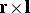. The components of the electric field vectors at a point,, in the beam can be written as(a1)

whereis the circular frequency,is time, and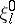andare (non-negative) amplitudes. One now defines the Stokes parameters by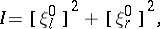(a2)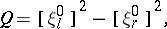(a3)(a4)(a5)

The end point of the electric vector at a point,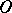, in the beam describes an ellipse, the so-called polarization ellipse, whose ellipticity and orientation with respect toandfollow from,and. If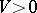, the electric vector atmoves clockwise, as viewed by an observer looking in the direction of propagation. Clearly, the following relation holds: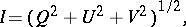(a6)

where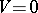for linearly polarized radiation and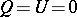for circularly polarized radiation.

In general, electromagnetic waves are not exactly monochromatic, but the amplitudesand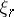, as well as the phase differences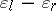, may vary slowly in time. In this case the Stokes parameters are defined as before, with one exception, namely time averages must be taken on the right-hand sides of (a2)–(a5). The polarization may now be partial and the beam can be decomposed in a completely unpolarized and a completely polarized beam. The orientation and shape of the polarization ellipse of the latter beam is again given by,and. The identity of (a6) is now replaced by the inequality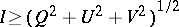(a7)

and the ratio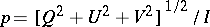is called the degree of polarization. For completely polarized radiation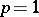, for partially polarized radiation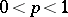, and for unpolarized (natural) radiation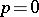.

The Stokes parameters can be combined into a column vector with elements,,, and, called a Stokes vector. Stokes vectors of constituent beams are added to obtain the Stokes vector of a composite beam if no interference effects occur. Optical devices and processes like scattering and absorption can be described by real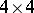(Mueller) matrices that transform the Stokes vectors of primary beams into those of secondary beams.

The Stokes parameters as defined above are one of many possible representations of polarized radiation, several of which are only slight modifications of each other (see, e.g., [a1]). Stokes parameters are also used in quantum mechanics in connection with polarization of elementary particles.

How to Cite This Entry:
Stokes parameters. Encyclopedia of Mathematics. URL: http://encyclopediaofmath.org/index.php?title=Stokes_parameters&oldid=13113
This article was adapted from an original article by C.V.M. van der MeeJ.W. Hovenier (originator), which appeared in Encyclopedia of Mathematics - ISBN 1402006098. See original article